- BetterExplained - https://betterexplained.com -

# Understanding Quake’s Fast Inverse Square Root

An article and research paper describe a fast, seemingly magical way to compute the inverse square root ($1/\sqrt{x}$), used in the game Quake.

I'm no graphics expert, but appreciate why square roots are useful. The Pythagorean theorem computes distance between points, and dividing by distance helps normalize vectors. (Normalizing is often just a fancy term for division.)

3D games like Quake divide by distance zillions (yes zillions) of times each second, so "minor" performance improvements help immensely. We don't want to take the square root and divide the regular way: exponentiation and division are really, really expensive for the CPU.

Given these conditions, here's the magic formula to get $1/\sqrt{x}$, as found in Quake (my comments inserted):


float InvSqrt(float x){
float xhalf = 0.5f * x;
int i = *(int*)&x;            // store floating-point bits in integer
i = 0x5f3759df - (i >> 1);    // initial guess for Newton's method
x = *(float*)&i;              // convert new bits into float
x = x*(1.5f - xhalf*x*x);     // One round of Newton's method
return x;
}


Yowza! Somehow, this code gets $1/\sqrt{x}$ using only multiplication and bit-shift operations. There's no division or exponents involved -- how does it work?

My Understanding: This incredible hack estimates the inverse root using Newton's method of approximation, and starts with a great initial guess.

To make the guess, it takes floating-point number in scientific notation, and negates & halves the exponent to get something close the the inverse square root. It then runs a round of Newton's approximation method to further refine the estimate and tada, we've got something near the inverse square root.

## Newton's Method of Approximation

Newton's method can be used to find approximate roots of any function. You can keep iterating the method to get closer and closer to the root, but this function only uses 1 step! Here's a crash-course on Newton's method (it was new to me):

Let's say you have a function f(x) and you want to find its root (aka where f(x) = 0). Let's call your original guess "g". Newton's method gives you a way to get a new, better guess for the root: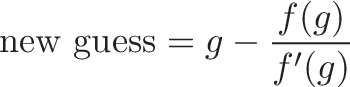$\displaystyle{\text{new guess} = g - \frac{f(g)}{f'(g)}}$

You can keep repeating this process (plugging in your new guess into the formula) and get closer approximations for your root. Eventually you have a "new guess" that makes f(new guess) really, really close to zero -- it's a root! (Or close enough for government work, as they say).

In our case, we want the inverse square function. Let's say we have a number $i$ (that's all we start with, right?) and want to find the inverse square root: $1/\sqrt{i}$. If we make a guess "x" as the inverse root, the error between our original number and our guess "x" is: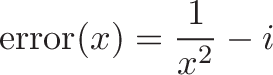$\displaystyle{\text{error}(x) = \frac{1}{x^2} - i}$

This is because x is roughly $1/\sqrt{i}$. If we square x we get $1/i$, and if we take the inverse we should get something close to $i$. If we subtract these two values, we can find our error.

Clearly, we want to make our error as small as possible. That means finding the "x" that makes error(x) = 0, which is the same as finding the root of the error equation. If we plug error(x) into Newton's approximation formula: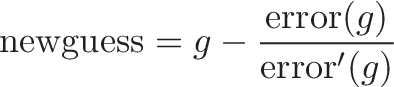$\displaystyle{\text{newguess} = g - \frac{\text{error}(g)}{\text{error}'(g)}}$

and take the proper derivatives: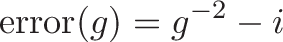$\displaystyle{\text{error}(g)= g^{-2} - i}$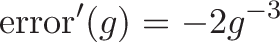$\displaystyle{\text{error}'(g)= -2g^{-3}}$

we can plug them in to get the formula for a better guess: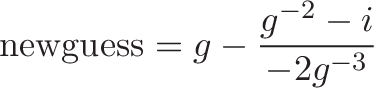$\displaystyle{\text{newguess} = g - \frac{g^{-2} - i}{-2g^{-3}} }$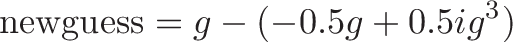$\displaystyle{\text{newguess} = g - (-0.5g + 0.5ig^3) }$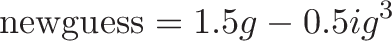$\displaystyle{\text{newguess} = 1.5g - 0.5ig^3}$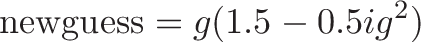$\displaystyle{\text{newguess} = g (1.5 - 0.5ig^2)}$

Which is exactly the equation you see in the code above, remembering that x is our new guess (g) and "xhalf" is half of the original value ($0.5 i$):

x = x*(1.5f - xhalf*x*x);

With this formula, we can start with a guess "g" and repeat the formula to get better guesses. Try this demo for using multiple iterations to find the inverse square:

In this demo, we start by guessing the square root is half the number: $\sqrt{n} \sim \frac{n}{2}$, which means $\frac{1}{\sqrt{n}} \sim \frac{2}{n}$. Running a few rounds of Newton's Method quickly converges on the real result. (Try n=2, 4, 10, etc.)

So my friends, the question becomes: "How can we make a good initial guess?"

## Making a Good Guess

What's a good guess for the inverse square root? It's a bit of a trick question -- our best guess for the inverse square root is the inverse square root itself!

Ok hotshot, you ask, how do we actually get $1/\sqrt{x}$?

This is where the magic kicks in. Let's say you have a number in exponent form or scientific notation: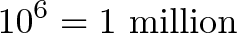$\displaystyle{10^6 = \text{1 million}}$

Now, if you want to find the regular square root, you'd just divide the exponent by 2: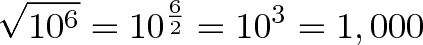$\displaystyle{\sqrt{10^6} = 10^{\frac{6}{2}} = 10^3 = 1,000}$

And if you want the inverse square root, divide the exponent by -2 to flip the sign: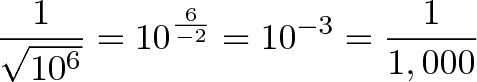$\displaystyle{\frac{1}{\sqrt{10^6}} = 10^{\frac{6}{-2}} = 10^{-3} = \frac{1}{1,000}}$

So, how can we get the exponent of a number without other expensive operations?

## Floats are stored in mantissa-exponent form

Well, we're in luck. Floating-point numbers are stored by computers in mantissa-exponent form, so it's possible to extract and divide the exponent!

But instead of explicitly doing division (expensive for the CPU), the code uses another clever hack: it shifts bits. Right-shifting by one position is the same as dividing by two (you can try this for any power of 2, but it will truncate the remainder). And if you want to get a negative number, instead of multiplying by -1 (multiplications are expensive), just subtract the number from "0" (subtractions are cheap).

So, the code converts the floating-point number into an integer. It then shifts the bits by one, which means the exponent bits are divided by 2 (when we eventually turn the bits back into a float). And lastly, to negate the exponent, we subtract from the magic number 0x5f3759df. This does a few things: it preserves the mantissa (the non-exponent part, aka 5 in: $5 \cdot 10^6$), handles odd-even exponents, shifting bits from the exponent into the mantissa, and all sorts of funky stuff. The paper has more details and explanation, I didn't catch all of it the first time around. As always, feel free to comment if you have a better explanation of what's happening.

The result is that we get an initial guess that is really close to the real inverse square root! We can then do a single round of Newton's method to refine the guess. More rounds are possible (at an additional computational expense), but one round is all that's needed for the precision needed.

## So, why the magic number?

The great hack is how integers and floating-point numbers are stored. Floating-point numbers like $5.4 \cdot 10^6$ store their exponent in a separate range of bits than "5.4". When you shift the entire number, you divide the exponent by 2, as well as dividing the number (5.4) by 2 as well. This is where the magic number comes in -- it does some cool corrections for this division, that I don't quite understand. However, there are several magic numbers that could be used -- this one happens to minimize the error in the mantissa.

The magic number also corrects for even/odd exponents; the paper mentions you can also find other magic numbers to use.

## Resources

There's further discussion on reddit (user pb_zeppelin) and slashdot: